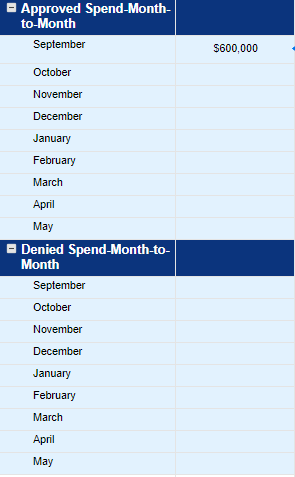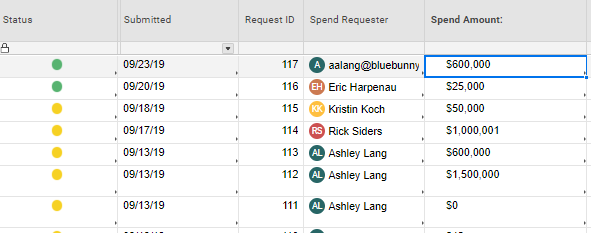# =SUMIF Month-to-Month

I am trying to create a graph for a Dashboard that will display the total amount of approved \$\$ within each month and total amount of denied \$\$ within each month. I will also need a rolling YTD total. So far this is what I have for the formula:

=SUMIFS({RSA Form Range 2}, {RSA Form Range 9}, "Green", {RSA Form Range 3}, DATE(2019, 9, 23))

I can not figure out to do a date range from 09/01/2019-09/30/2019 to show the total amount of either denied/approved \$\$'s.

Attached is the sheet I am getting the data for the sheet metrics to be used on a dashboard.• Instead of trying to specify the first and last day of the month, you can just specify the month. I also suggest specifying the year in case you have multiple years on one sheet.

=SUMIFS({RSA Form Range 2}, {RSA Form Range 9}, "Green", {RSA Form Range 3}, AND(IFERROR(MONTH(@cell), 0) = 9, IFERROR(YEAR(@cell), 0) = 2019))

• That worked perfectly! I kept trying to do a date range and that kept coming back #UNPARSABLE or gave me an error. So i reverted back to just specifying a certain date.

Thank you very much!

• I'm not sure I follow...

Using MONTH(@cell) = 9 and YEAR(@cell) = 2019 is what gives you your date range. It specifies to look at all dates that are greater than or equal to the 1st of the month and less than or equal to the end of the month (within the specific year of course). You shouldn't have to enter a specific date into the formula.

• Oh no, I was saying the formula you gave to me worked perfectly for what I was looking for. What I was doing prior to posting in the community, was trying to put date ranges into the formula and it never worked, so I had reverted back to a specific date when I posted for help.

• Ooooh. Ok. Haha. Well I'm glad I could help.• I am trying to figure out what I am doing wrong.  My goal is to get a total of open and closed items each month. Here is the formula that I am using:

=SUMIFS({National Alliance Operation Inquiries Range 1}, {National Alliance Operation Closed Inquiri Range 1}, AND(IFERROR(MONTH(@cell, 0) = 4, IFERROR(YEAR(@cell), 0) = 2020))

Receiving error : #INCORRECT ARGUMENT SET

• @Beronica Muller You are going to want to use a COUNTIFS instead and remove that first range. You also have a missing parenthesis in your IFERROR(MONTH portion. Try this...

=COUNTIFS({National Alliance Operation Closed Inquiries Range 1}, AND(IFERROR(MONTH(@cell), 0) = 4, IFERROR(YEAR(@cell), 0) = 2020))

• @Paul Newcome Should I remove the first range?  I wanted to get a total count of open and closed items which are from two separate sheets. Thanks for assisting with this.

Also, I am receiving an error message when I enter the formula that you provided.

=COUNTIFS({National Alliance Operation Closed Inquiries Range 1}, AND(IFERROR(MONTH(@cell), 0) = 4, IFERROR(YEAR(@cell), 0) = 2020)) #UNPARSEABLE

• If you are going to reference two separate sheets, you are going to need two add two separate COUNTIFS together.

=COUNTIFS({Sheet 1}, .................) + COUNTIFS({Sheet 2}, ...................)

How are you creating your cross sheet reference?

• We can keep with separate sheets if it is easier. Once this formula works I can create the same formula from a seperate reference sheet. I'm creating my cross reference sheets by selecting the 'reference another sheet' link from the help card once I begin typing the formula. From there, I select the column from which I want the data.

• Ok. So it sounds like you are following the correct steps to create the cross sheet references.

I am not seeing any syntax issues with the formula you posted either.

Can you provide a screenshot of the formula exactly as it is in the sheet similar to how I have my screenshot below? Being able to see the formula in sheet may show something that is missing when you type it out here.

## Help Article Resources

Want to practice working with formulas directly in Smartsheet?

Check out the Formula Handbook template!